# PyTorch 04：计算图与动态图机制

## PyTorch 学习笔记

Posted by YEY on December 8, 2020

# Lecture 04 计算图与动态图机制

## 1. 计算图

• 结点表示 数据，例如：向量、矩阵、张量。
• 边表示 运算，例如：加、减、乘、除、卷积等。

• $a = x+w$
• $b=w+1$
• $y=a*b$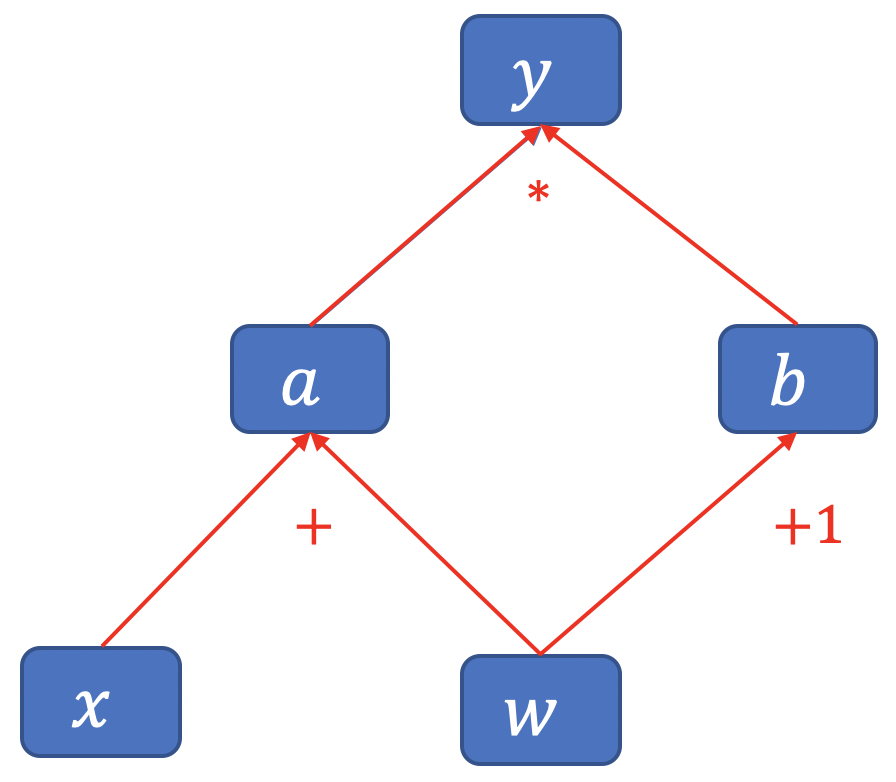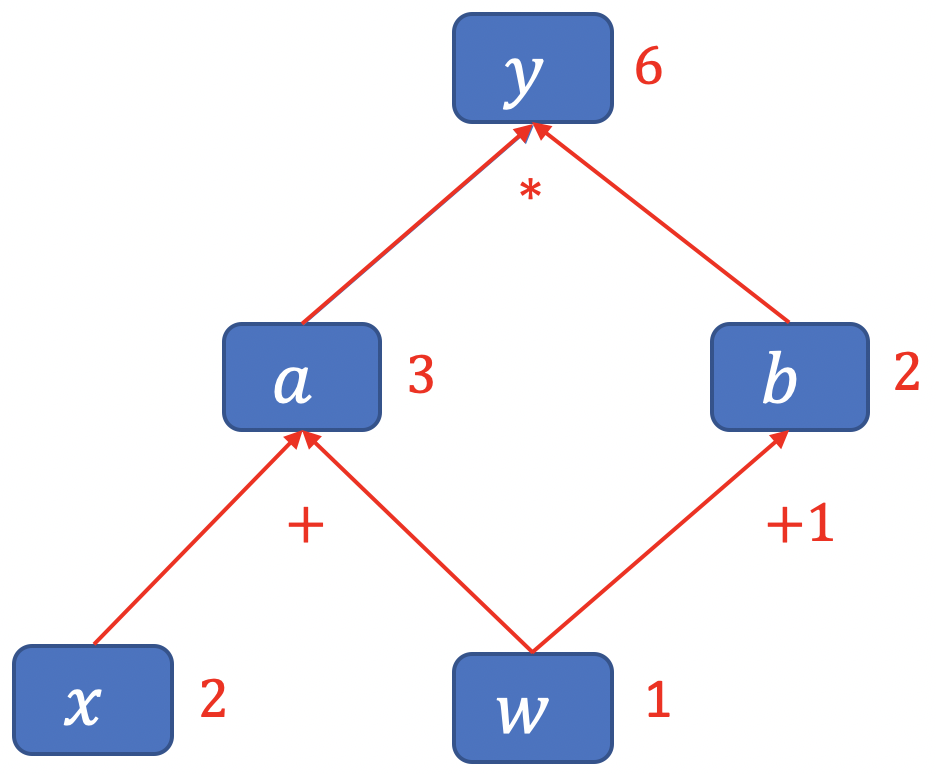$y=(x+w) * (w+1)$
• $a = x+w$
• $b=w+1$
• $y=a*b$
\begin{align} \dfrac{\partial y}{\partial w} &= \dfrac{\partial y}{\partial a} \dfrac{\partial a}{\partial w} + \dfrac{\partial y}{\partial b} \dfrac{\partial b}{\partial w} \\[2ex] &= b * 1+ a * 1 \\[2ex] &= (w+1) + (x + w) \\[2ex] &= 2*w + x + 1 \\[2ex] &= 2*1 + 2 + 1 = 5 \end{align}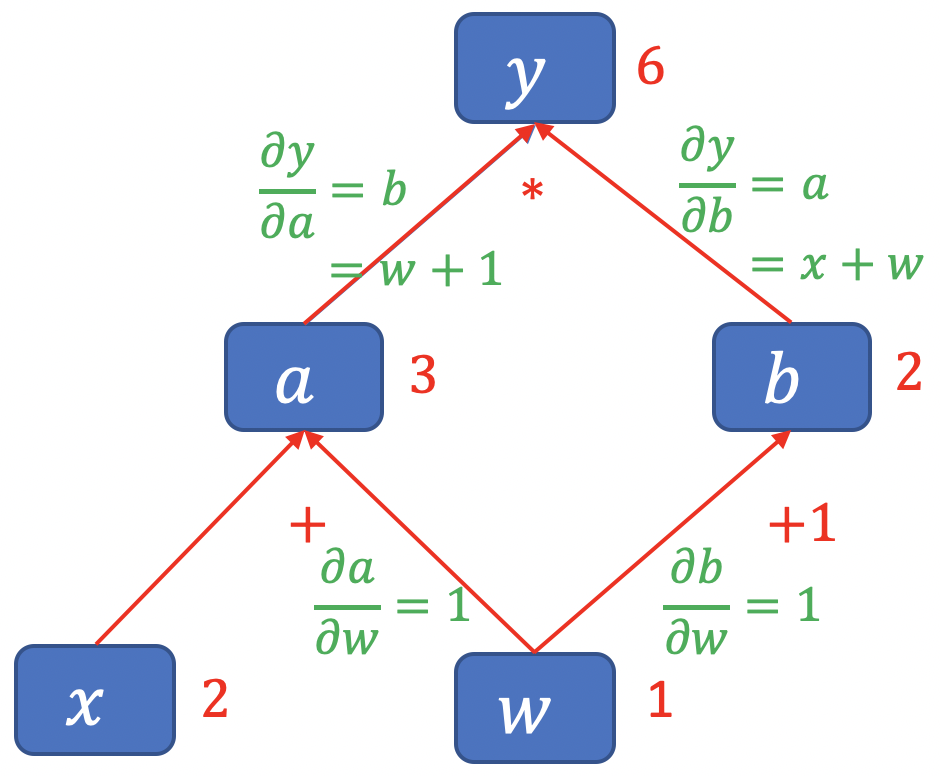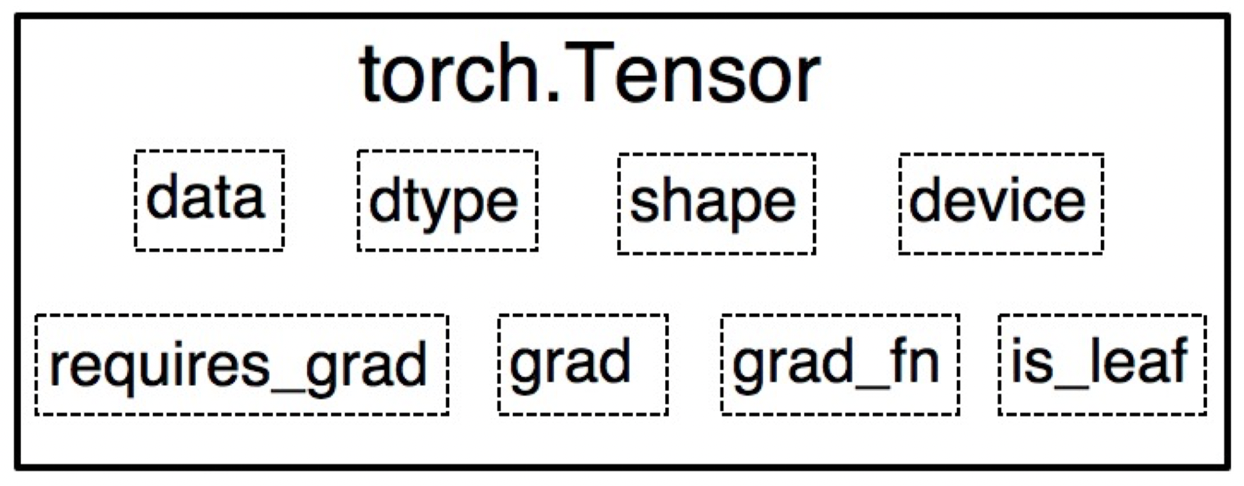• y.grad_fn = <MulBackward0>
• a.grad_fn = <AddBackward0>
• b.grad_fn = <AddBackward0>

Python 代码示例

1
2
3
4
5
6
7
8
9
10
11
12
13
14
15
16
17
18
19
20
21
22
23
24
25
26
27
28
29
import torch

# 叶子结点

# 非叶子结点
y = torch.mul(a, b)

# PyTorch 中，为了节省内存，在计算完成后会丢弃非叶子结点的梯度值，即为 None；

# 反向传播
y.backward()

# 查看叶子结点
print("is_leaf:\n", w.is_leaf, x.is_leaf, a.is_leaf, b.is_leaf, y.is_leaf)

# 查看梯度



1
2
3
4
5
6
7
tensor([5.])
is_leaf:
True True False False False
tensor([5.]) tensor([2.]) tensor([2.]) tensor([3.]) tensor([1.])
None None <AddBackward0 object at 0x1146d1518> <AddBackward0 object at 0x1146d1550> <MulBackward0 object at 0x1146d15c0>


## 2. 动态图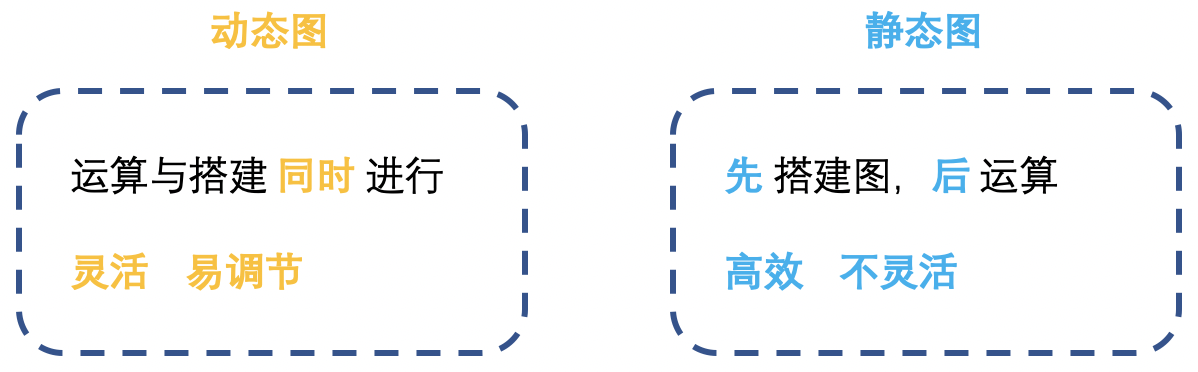## 3. 总结本作品采用知识共享署名-非商业性使用-相同方式共享 4.0 国际许可协议进行许可。 欢迎转载，并请注明来自：YEY 的博客 同时保持文章内容的完整和以上声明信息！